# BCK-algebra

Algebras originally defined by K. Iséki and S. Tanaka in [a7] to generalize the set difference in set theory, and by Y. Imai and Iséki in [a5] as the algebras of certain propositional calculi. A BCK-algebra may be defined as a non-empty setwith a binary relationand a constant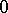satisfying the following axioms:

1);

2);

3);

4)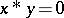and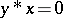imply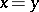;

5)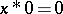implies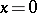;

6)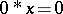for all. A partial ordercan then be defined by putting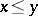if and only if. A very useful property is.

A BCK-algebra is commutative if it satisfies the identity(cf. also Commutative ring). In this case,, the greatest lower bound ofand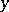under the partial order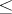. The BCK-algebra is bounded if it has a largest element. Denoting this element by, one has, the least upper bound ofand. In this case,is a distributive lattice with boundsand. A BCK-algebra is positive implicative if it satisfies the identity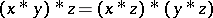. This is equivalent to the identity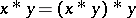.is called implicative if it satisfies the identity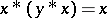. Every implicative BCK-algebra is commutative and positive implicative, and a bounded implicative BCK-algebra is a Boolean algebra.

An ideal of a BCK-algebra is a non-empty setsuch that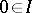and if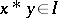andimply. The ideal is implicative if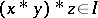and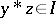imply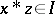. It is known that always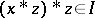. Note that in a positive implicative BCK-algebra, every ideal is implicative. Implicative ideals are important because in a bounded commutative BCK-algebra they are precisely the ideals for which the quotient BCK-algebras are Boolean algebras. Here, ifis an ideal in a BCK-algebra, one can define a congruence relation inby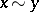if and only ifand. The setof congruence classes then becomes a BCK-algebra under the operation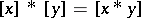, withas the constant and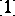as the largest element if there exists a largest element. Some, but not all, of the well-known results on distributive lattices and Boolean algebras hold in BCK-algebras, in particular in bounded commutative BCK-algebras. For example, the prime ideal theorem holds for bounded commutative BCK-algebras, that is, ifis an ideal and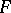is a lattice filter such that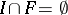, then there exists a prime ideal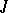such that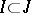and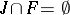. Here, "prime ideal" simply means that if it contains, then it contains eitheror.

Some of the homological algebra properties of BCK-algebras are known, see [a2]. There is also a close connection between BCK-algebras and commutative-groups with order units (cf.-group). Recall that an elementin the positive cone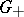of a commutative-groupis an order unit if for each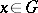one has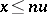for some integer. Let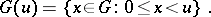For, let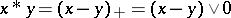. Thenis a commutative BCK-algebra.

Fuzzy ideals of BCK-algebras are described in [a3] and [a4]. General references for BCK-algebras are [a6] and [a7].

How to Cite This Entry:
BCK-algebra. C.S. Hoo (originator), Encyclopedia of Mathematics. URL: http://encyclopediaofmath.org/index.php?title=BCK-algebra&oldid=16457
This text originally appeared in Encyclopedia of Mathematics - ISBN 1402006098